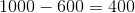# PSAT Math : How to find the volume of a cube

## Example Questions

2 Next →

### Example Question #11 : How To Find The Volume Of A Cube

A cube is inscribed inside a sphere of radius 1 such that each of the eight vertices of the cube lie on the surface of the sphere.  What is the volume of the cube?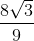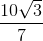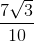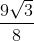Explanation: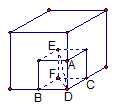To make this problem easier to solve, we can inscribe a smaller square in the cube.  In the diagram above, points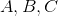are midpoints of the edges of the inscribed cube.  Therefore point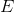, a vertex of the smaller cube, is also the center of the sphere.  Pointlies on the circumference of the sphere, so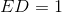.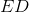is also the hypotenuse of right triangle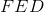.  Similarly,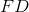is the hypotenuse of right triangle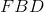.  If we let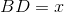, then, by the properties of a right triangle,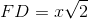.

Using the Pythagorean Theorem, we can now solve for: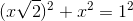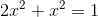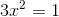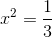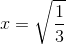Since the side of the inscribed cube is, the volume is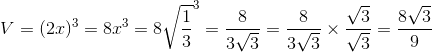.

### Example Question #12 : How To Find The Volume Of A Cube

If a cube has a surface area of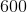, what is the difference between the volume of the cube and the surface area of the cube?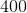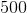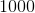If the surface area is, then the area of one face must be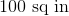. Therefore, the length of one edge must be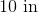This means that the volume of the cube is. We can now solve with: